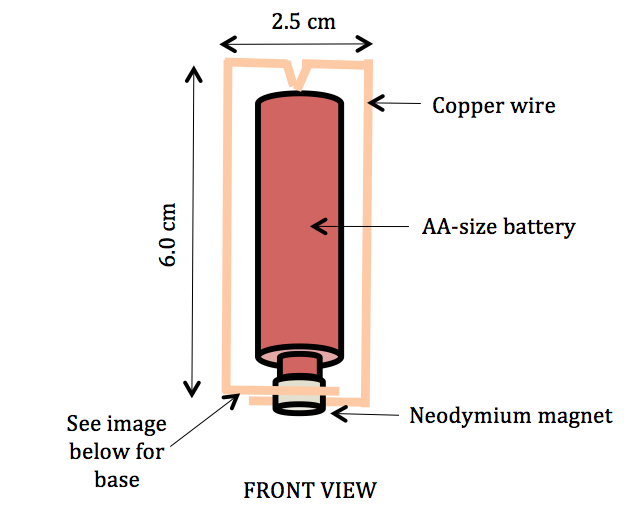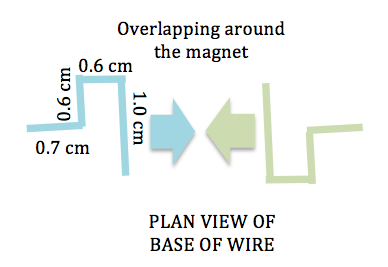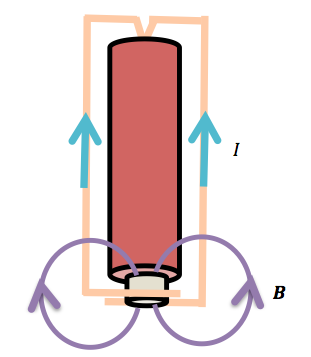# electromagnetism

## Homopolar Motor 2

This video demonstrates how a simple homopolar motor is made using a screw and a small neodymium magnet. The simplest possible motor one can make, it can be used to teach concepts at various levels. For lower secondary students, they can learn about conversion of energy forms while upper secondary students can learn about magnetic forces and Fleming’s left-hand rule.

Materials

1. 10 cm wire
2. 1.5 V battery
3. iron screw
4. neodymium magnet

Procedure

1. Attach the neodymium magnet to the head of the screw.
2. Attach the tip of the screw to one end of the battery such that the screw hangs below the battery. The screw will remain attached to the battery as the magnetic force from the neodymium holds them together.
3. Hold one end of the wire on the top terminal of the battery and allow the other end of the wire to touch the side of the screw or the magnet. Watch the screw spin.

## Electromagnetism Lecture

I enjoy lecturing on topics like Superposition and Electromagnetism in the GCE A-level syllabus as they lend themselves well to the use of fun demonstrations that I can perform in front of the audience.

One of the recent demonstrations that I did was to demonstrate the measurement of the magnetic force acting on a wire and to show that the force can be inverted when the current is reversed. The magnitude of the force can be shown to be consistent with the relationship $$F = BIl sin theta$$, where $$B$$ is the magnetic flux density, $$I$$ is the current within the wire, $$l$$ is the length of the wire and $$theta$$ is the angle between the wire and the magnetic field. This can be illustrated by independently varying one of the 4 variables and observing the change in force.

The setup is also a good for a demonstration to illustrate Fleming’s Left-Hand Rule.

For more details, visit http://www.scienses.com/measuring-the-force-on-a-current-carrying-conductor/.

Meanwhile, here’s a video I made to show what I did:

## Measuring the Force on a Current-Carrying Conductor

The force acting on a current-carrying conductor is given by $$F =BIl sin theta$$ where B is the magnetic flux density, I is the current, l is the length of the wire and $$theta$$ is the angle between the current and the magnetic field. It can be measured in directly using a weighing scale as described below:

Materials

1. An electronic weighing scale
2. Two neodymium magnets
3. Plasticine
4. Batteries
5. 40 cm long wire

Procedure

1. Place some plasticine between two neodymium magnets to hold them together with each magnet having a different pole facing up (north for one and south for the other).
2. Place the magnets on the weighing scale.
3. Connect the 40 cm wire across the opposite terminals of the batteries.
4. Hold the wire horizontally and place it between the two magnets so that the current runs perpendicularly to the magnetic field lines.
5. Using Fleming’s left-hand rule, one can predict whether the measured weight will increase or decrease. If the magnetic force acting on the wire is upward, by Newton’s 3rd law, the reaction force acting on the magnets is downward and the measured weight will increase, and vice versa.
6. Flipping the current around in the opposite direction will yield opposite results.

## Homopolar Motor 1

A homopolar motor is a simple electric motor that does not require the use of a commutator. The electric current flows in a fixed direction within the wires of the motor. The following are instructions on how to construct this simple teaching tool that can be used to demonstrate how a motor works, as well as teach concepts such as Fleming’s left-hand rule and $$\mathbf{F}= I\mathbf{l \times B}$$.

Materials

1. Copper wire (about 22 cm)
2. Small neodymium magnets (1 or 2)
3. 1.5 V AA-size battery
4. Base with either another magnet or a iron surface, such as the head of an iron nail

Procedure

1. Make a V-shaped bend in the middle of the copper wire, with about 0.5 cm on both sides of the V-shape. Bend the copper wire into a rectangular loop using the dimensions shown below.2.Tip: You may use the edge of a wooden block as a guide to bend the copper wires at right angles. A pair of wooden blocks can also be used to flatten the rectangular loop if you press them together tightly with the loop in between.
3. Mount the neodymium magnet(s) onto the magnet or iron base.
4. Hook the wires at the base around the magnets.
5. Place the AA-sized battery with the protruding end on the magnet(s).
6. Complete the electric circuit by placing the V-shaped end of the rectangular loop onto the flat end of the battery and watch the loop spin.
7. Be careful not to keep the current flowing for too long as the battery and wire can get very hot.

Science Explained

1. A force acts on a current if it is placed in a magnetic field. This force is what causes the motor to spin about its axis.
2. To apply Fleming’s left hand rule, observe from the diagram below how the magnetic field bends around the magnet and its direction with respect to the direction of current flow. How do you think the loop will spin?If you are having difficulty making this version of the homopolar motor, try the other design for the homopolar motor made using a screw.